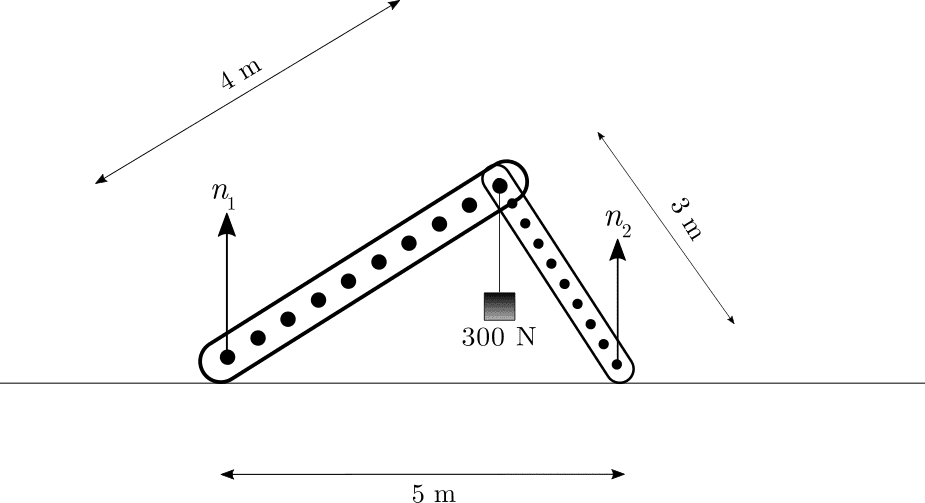# Normal reactions at the bases of two light supports

Homework Statement:
Shown in the figure below are two light plastic supports pinned at their top. One of the supports is 4 m long while the other is 3 m long and they are arranged to form a right angled triangle with their base 5 m. A mass of 300 N is hung from the pin at the top. If the ground is frictionless, calculate the reactions ##n_1## and ##n_2## at the bases of the two supports.
Relevant Equations:
For equilibrium, ##\Sigma \vec F = 0## for the system as a whole and ##\Sigma \vec \tau = 0## for any point on the system where torque ##\vec \tau = \vec r \times \vec F##.For equilibrium, using ##\Sigma \vec F = 0##, we get ##n_1 + n_2 = 300\; \text{N}##.

Taking the system as a whole and applying ##\Sigma \vec \tau = 0## about the hinge (pin) at the top from where the load is hung, we get ##n_1 \times (0.8) \times 4 = n_2 \times (0.6) \times 3##, by taking those components of the normal forces perpendicular to the supports and using trigonometry.

Hence, ##3.2 n_1 = 1.8 n_2 \Rightarrow n_2 = \frac{16}{9} n_1##.

Thus, going to the earlier equation, ##n_1 + \frac{16}{9} n_1 = 300 \Rightarrow \frac{25}{9} n_1 = 300 \Rightarrow \boxed{n_1 = 108 \; \text{N}}##.
Also, ##\boxed{n_2 = 192 \; \text{N}}##.

Is this right?

Even if it is, is there a physical explanation as to why ##n_2 > n_1##? Can we say it is because ##n_2## has a smaller lever arm than ##n_1## and therefore has to be greater in order to nullify the torque produced by ##n_1## about the hinge?

## Answers and Replies

hutchphd
Science Advisor
Homework Helper
Or that the mass of the "object" is horizontally closer to point 2 which therefore sees more force. There are a hundred ways to say it! Very nicely done.

haruspex
Science Advisor
Homework Helper
Gold Member
2020 Award
Another approach is to take moments about each support point in turn and dispense with the vertical force balance equation. This has the slight advantage of avoiding having to solve a pair of simultaneous equations, but it is more useful when you only need to find one reaction force.

PhanthomJay
Science Advisor
Homework Helper
Gold Member
Where did this problem come from? If there is a true pin at the Top, and if the supports at their base sit on frictionless ground, this system will instantly collapse.